## Average For SBI PO set 2

1. In a class of 52 students, the number of boys is two less than the number of girls. Average weight of the boys is 42 kg, While the average weight of all the 52 student is 40 kg. Approximately what is the average weight of the girls?

a) 41kg

b) 39kg

c) 40 kg

d) 38kg

e) 42kg

d) 38kg

Explanation:

let the number of boys=x; and

number of girls = y

Now, (y-2)=x and x+y=52

y-2+y=52

2y=54

Y=27

X=25

Let the average weight of the girls be ‘a’.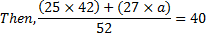Or 27×a=(52×40)-(25×42)

Or 27×a=2080-1050

a =1030/27

=38.148

=38

2. The average Income of P, Q, R, S and T is Rs 15000. The average income of P &Q is Rs 12000 and the average income of R&S is Rs 16000, find the average income of T.

a) Rs 19000

b) Rs 20000

c) Rs 23000

d) Rs 12000

e) none of these

a) Rs 19000

Explanation:

sum of the incomes of P, Q, R, S and T=15000× 5=75000

sum of the incomes of P, Q=2×12000=24000

sum of the incomes of R& S=2×16000=32000

Average income of T

= (P+Q+ R+S+T)-(P+Q)-(R+S)

= 75000-24000-32000=19000

3. The average number of coins with A, B, C and Dare 5. The average number of coins with D, E and F are 7. Find the number of coin with all the 6 people is 6.

a) 3

b) 5

c) 4

d) 7

e) 8

b) 5

Explanation:

Total number of coins with A, B,C,& D=5×4=20

Total number of coins with D,E&F=3×7=21

Total number of coins with A, B, C, D, E & F=6×6=36

Number of coins with D=(A+B+C+D)+(D+E+F)-(A+B+C+D+E+F)

=20+21-36=5

4. Samdha travels 1/3rd of distance from delhi to Jaipur at speed of 80km, nest 1/3rd distance at speed of 100kn. The average speed of Samdha for the entire journey is:

a) 90km

b) 89.25km

c) 90.16km

d) None of these

e) 39.67km

b) 89.25km

Explanation:

Since distance travelled at different speed is same, we can use the short cut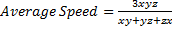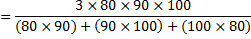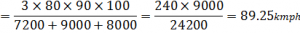5. Gabbar Singh travelled from Ramgarh to Vijaygarh at speed of 60km and fromVijaygarh to laxmangarh at speed of 40km. then he returned back from laxmangarhto Ramgarh to meet Basanti, at speed of 80 km. if the distance between Ramgarh and Vijaygarh is same as distance between Vijaygarh and laxmangarh, then find the average speed of Gabbar Singh for the whole journey:

a) 48km

b) 60km

c) 56 km

d) None of these

e) 67km

b) 60km

Explanation:

The average speed of gabbarsingh from Ramgarh to Laxmangath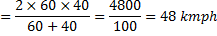[Because distance from Ramgarh to Vijaygarh and from Vijaygarh to Laxmangathis same].

He is travel from Laxmangath  toRamgarh at speed of 80km

The average speed of entire journey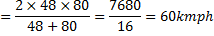6. The average income of all the employees of ABC Ltc is Rs 12000 in 1999. In the year 2000, the company gave a bonus of Rs 2000 to each of the employees,then the new average is

a) Rs 12000

b) Rs 1400

c) Rs 14000

d) None of these

e) Rs 120000

c) Rs 14000

Explanation:

Since all the employees are given a bonus of Rs 2000, hence the average would also increase by Rs 2000.

7. The average expenditure of P, Q and R is Rs 5000 per month. Also, the average expenditure of Q, R and S is Rs 7000 per month. If the average expenditure of S is thrice of that of P then the average expenditure of Q and R is:

a) Rs 6000

b) Rs 3000

c) Rs 5000

d) Rs 4000

e) none of these

a) Rs 6000

Explanation:

Total expenditure of P, Q & R=3×5000=15000

Total expenditure of Q, R & S =3×7000=21000

S-P=21000-15000=6000

Also, we are given S=3P. so 3P-P =6000 & P=3000

Total expenditure of Q & R=15000-3000=12000

Average expenditure of Q& R=12000/2 =6000

8. The average age of 8 person is increased by 2 years when one of them, whose age is 24 years id replaced by a new person. The age of new person is

a) 42 years

b) 40years

c) 38years

d) 45 years

e) None of these

b) 40years

Explanation:

The average age of 8 person is increased by 2i.e sum of the age would increase by 16. Age of the new person would be 16 years more than age of the person replaced, so age3 of new person =24+16=40 years

9. The average age of five officers in a department is 32 years. If the age of their supervisor is added, the average age increased by 1. What is the supervisor’s age?

a) 32years

b) 48years

c) 38years

d) 42 years

e) None of these

c) 38years

Explanation:

The new average is increased by 1 year hence sum of the age would have increased by 6years. Age of supervisor =32+6=38 years

10. In a one-day cricket match the captain of one of the teams scored 30 runs more than the average runs scored by the remaining six batsmen of that team who batted in the match. If the total runs scored by all the batsman of that were 310, how many runs did the captain score?

a) 60

b) 70

c) 50

d) Cannot be determined

e) None of these

b) 70

Explanation:

Let the average runs scored by 6 batman =x

Total runs scored by them =6x

Then, 6x+x+30=310

7x=280

X=40

Score of captain=40+30=70